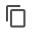EXPAND ALL
• Home
Open Source Docs

# Tutorial #1: Write your first PxL scriptThis tutorial series demonstrates how to write a PxL script to analyze the volume of traffic coming in and out of each pod in your cluster (total bytes received vs total bytes sent).

In Part 1 of this tutorial, we will write a very basic PxL script which simply queries a table of traced network connection data provided by Pixie's no-instrumentation monitoring platform.

## The most basic PxL script1. Create a new PxL file called `my_first_script.pxl`:
`touch my_first_script.pxl`
1. Open this file in your favorite editor and add the following lines. To copy the code, hover over the top-right corner of the code block and click the copy icon.
`1# Import Pixie's module for querying data2import px34# Load the last 30 seconds of Pixie's `conn_stats` table into a Dataframe.5df = px.DataFrame(table='conn_stats', start_time='-30s')67# Display the DataFrame with table formatting8px.display(df)`

On `line 2` we import Pixie's `px` module. This is Pixie's main library for querying data.

Pixie's scripts are written using the Pixie Language (PxL), a DSL that follows the API of the the popular Python data processing library Pandas. Pandas uses DataFrames to represent tables of data.

On `line 5` we load the last 30 seconds of data from the `conn_stats` table into a DataFrame.

The `conn_stats` table contains high-level statistics about the connections (i.e. client-server pairs) that Pixie has traced in your cluster.

On `line 8` we display the table using `px.display()`.

1. Run this script using Pixie's Live CLI:
`px live -f my_first_script.pxl`

Your CLI should output something similar to the following table:

This PxL script outputs a table of data representing the last 30 seconds of the traced client-server connections in your cluster. Columns include:

• `time_`: Timestamp when the data record was collected.
• `upid` An opaque numeric ID that globally identifies a running process inside the cluster.
• `remote_addr`: IP address of the remote endpoint.
• `remote_port`: Port of the remote endpoint.
• `addr_family`: The socket address family of the connection.
• `protocol`: The protocol of the traffic on the connections.
• `role`: The role of the process that owns the connection (client=1 or server=2).
• `conn_open`: The number of connections opened since the beginning of tracing.
• `conn_close`: The number of connections closed since the beginning of tracing.
• `conn_active`: The number of active connections.
• `bytes_sent`: The number of bytes sent to the remote endpoint(s).
• `bytes_recv`: The number of bytes received from the remote endpoint(s).

## (Optional) Running px/schemasYou can find the `conn_stats` column descriptions as well as descriptions for all of the data tables provided by Pixie in the data table reference docs or by running the pre-built `px/schemas` script:

1. Exit the Live CLI using `ctrl+c`

2. Run the `px/schemas` script:

`px live px/schemas`
1. Use the keyboard arrows to scroll down through the output table until you reach `conn_stats` in the `table_name` column. You should see all of the columns available in the `conn_stats` table listed with their descriptions.

## (Optional) More fun with DataFramesDataFrame initialization supports `end_time` for queries requiring more precise time periods. If an `end_time` isn't provided, the DataFrame will return all events up to the current time.

`1import px23df = px.DataFrame(table='conn_stats', start_time='-60s', end_time='-30s')45px.display(df)`

You can drop columns using the `df.drop()` command.

`1import px23df = px.DataFrame(table='conn_stats', start_time='-30s')45# Drop select columns6df = df.drop(['conn_open', 'conn_close', 'bytes_sent', 'bytes_recv'])78px.display(df)`

Alternatively, you can use keep to return a DataFrame with only the specified columns. This can be used to reorder the columns in the output.

`1import px23df = px.DataFrame(table='conn_stats', start_time='-30s')45# Keep only the select columns6df = df[['remote_addr', 'conn_open', 'conn_close']]78px.display(df)`

If you only need a few columns from a table, use the DataFrame's `select` argument instead.

`1import px23# Populate the DataFrame with only the select columns from the `conn_stats` table4df = px.DataFrame(table='conn_stats', select=['remote_addr', 'conn_open', 'conn_close'], start_time='-30s')56px.display(df)`

To filter the rows in the DataFrame by the `role` column:

`1import px23df = px.DataFrame(table='conn_stats', start_time='-30s')45# Filter the results to only include rows whose `role` value equals 1 (connections traced on the client-side)6df = df[df.role == 1]78px.display(df)`

If you want to see a small sample of data, you can limit the number of rows in the returned DataFrame to the first n rows (line 4).

`1import px23df = px.DataFrame(table='conn_stats', start_time='-30s')45# Limit the number of rows in the DataFrame to 1006df = df.head(100)78px.display(df)`

## ConclusionCongratulations, you built your first script!

In Tutorial #2, we will expand this PxL script to produce a table that summarizes the total amount of traffic coming in and out of each of the pods in your cluster.

This video summarizes the content in part 1 and part 2 of this tutorial: